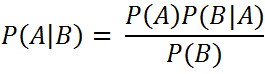`  I request GUEST WRITERS for this web site. Anyone interested please contact me at saravu2k2013@gmail.com Thanks  `
Showing posts with label Data Structures Programs. Show all posts
Showing posts with label Data Structures Programs. Show all posts

## Binary search algorithm implementation using C program

### Program:

/* recursive binary search*/
#include<stdio.h>

int bsearch(int [ ],int, int, int); //function declaration

int main( )
{
int a, i, low_index, high_index;
int position, total_keys, key_to_find
printf("\nEnter the total number of values:");
scanf("%d", &total_keys);

for(i = 0; i < total_keys; i++)
{
printf("\nEnter the key elements %d  : ", i);
scanf("%d", &a[i]);
}
printf("\nEnter the key to search : ");
scanf("%d", &key_to_find);
low_index = 0;
high_index = n-1;
//the following line calls the function bsearch with parameters
position = bsearch(a, key_to_find, low_index, high_index);
if(position!=-1)
printf("Search successful, element found at position %d", position);
else
getch();
}

int bsearch(int a[ ], int k, int lb, int ub)
{
int mid;
while(ub >= lb) //repeat this loop while upper bound >= lower bound
{
mid=(lb+ub)/2; //finds new middle index in each iteration
if(k < a[mid]) //if the key_to_find is smaller than middle element of array,
ub = mid-1; //set the new upper bound as middle-1
else if(k > a[mid]) //if key_to_find is larger than middle
lb = mid+1; //set the new lower bound as middle+1
else if(k == a[mid]) //if key_to_find is equal to middle element
return(mid); //return the middle element as result
return (bsearch(a,k,lb,ub)); //if none of the above holds, call bsearch (recursive)
}
return -1; //if the key_to_find is not in the list, return -1 to tell ‘NOT FOUND’
}

**************

binary search program in C
binary search algorithm using recursive functions in C
binary search divide and conquer algorithm
binary search simple program in C

## Linear/Sequential Search program in C

Program:

#include <stdio.h>
#include<conio.h>

int linear (int a[], int tot_keys, int k);
int main()
{
int a;
int n,i,key;
int flag=0, found_at;
printf ("\n Enter the number of elements : ");
scanf ("%d", &n);

for(i=0; i<n; i++)   //to read list of keys
{
printf("\n Enter the key %d:", i);
scanf("%d", &a[i]);
}
printf("\nEnter the data to be searched for:");
scanf("%d", &key);

//Searching...
//following line calls the function linear with the parameters array of keys (a), total //keys (n) in the array, and the key (key) to be searched. The result returned by the //function stored in variable ‘found_at’.
found_at = linear(a, n, key);
if(found_at!=-1)
printf("\nData is present at position %d", found_at);
else
printf("\nData not present");
getch();
}
int linear(int a[], int tot_keys, int k)
{
int i, position = -1;
for(i=0; i < tot_keys; i++) //this loop executes the number of keys time
if(a[i]==k) //checks if the key at position i is equal to key to be searched
position = i; //we remember the position of key in array
return position;
}

***************

Linear search program in C
Sequential search program in C

## C program for implementing balanced parentheses using Stack ADT using array explained, How to implement stack ADT using array for balanced parentheses? How to implement stack data structure using linear array in C?

### Implementation of balanced parentheses using stack with array

/* This program implements balanced parentheses using stack with array. It handles [], (), and {} brackets. In the input string, if the brackets are not balanced, then the program shows “Unbalanced” otherwise shows “Balanced” as result.
Working
The program scans the input string from left to right character by character. If the scanned character is opening bracket, then inserts the character on to the stack. If the scanned character is closing symbol ( ‘)’, ‘]’, ‘}’ ), then the program pops the top element. */

#include <stdio.h>
#include <conio.h>
#define max 50 //to declare a constant variable (a macro) with a value
int main()
{
char stk[max],exp;
int top,i;     //declares a variable top to point to the top element of stack
top = -1;     //initialize the top of stack with -1, that is, empty stack.
printf("\nEnter an infix expression ");
gets(exp);
for(i=0; exp[i] != '\0'; i++) //’\0’ used to represent NULL
{
//checks whether the input character is any of the opening symbol
if( exp[i]=='(' || exp[i] =='[' || exp[i] == '{' )
{
top++; //if the input is opening bracket, then top is incremented to 1
stk[top]= exp[i]; //pushes the opening bracket into stack
}
else
if ( exp[i] == ')' ) //if the input is ), then we check for the top element
{
if( stk[top] == '(' ) //if the top of stack is (, then pop the top element
{
top--; //pop is achieved by decrementing the top variable value
}
else
{
printf("Unbalanced exp"); //if top element is other than (, then print this
getch();
exit(0); //in case of unbalanced, terminate the program
}
}
else
if ( exp[i] == ']' ) //the above stated thing is checked for ] square bracket
{
if( stk[top] == '[' )
top--;
else
{
printf("Unbalanced exp");
getch();
exit(0);
}
}
else
if ( exp[i] == '}' ) //the above stated thing is checked for } curly brace
{
if( stk[top] == '{' )
top--;
else
{
printf("Unbalanced exp");
getch();
exit(0);
}
}
} // for
if( top == -1 )
printf("Exp is balanced");
else
printf("Exp is not balanced");
getch();
} // main

***************

### Simple introduction to Naive Bayes classifier

Simple introduction to Naive Bayes classifier What is Naive Bayes Classifier? A Naive Bayes classifier is a probabilistic classifier ...